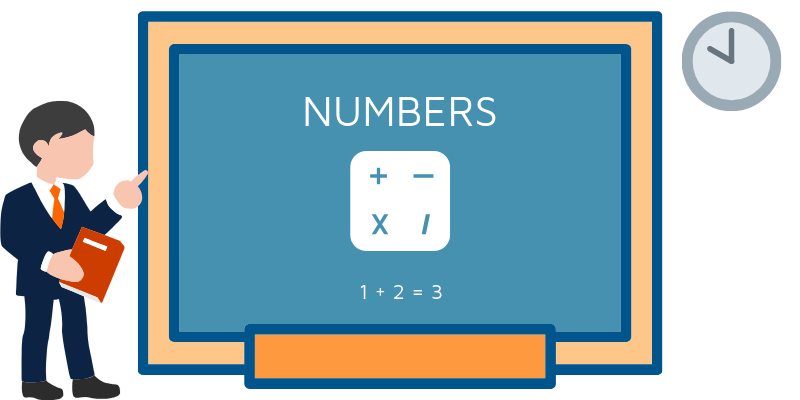English XP# Numbers

## Simple Numbers

Learning the numbers in English is done fairly early on. Counting up to a hundred is fairly simple even if you haven’t been learning English for a long time. It get’s a little more difficult however when we go higher. Let’s have a recap of the bigger numbers as well as clarifying a few things.

In British English, whenever there is a number after a hundred, we usually join it with and. You will often hear natives shorten this to an n sound.

805 = eight hundred and five

The same is done with thousands and smaller numbers. However and is never put between thousands and hundreds.

1,807 = one thousand, eight hundred and seven

In American English the ‘and’ is often dropped. (e.g “nine hundred ninety nine” not “nine hundred and ninety nine”). This depends on where (and whom) you speak to though.

## Simple Number Examples

Here are a few examples showing where the and is in bigger numbers:

104 = one hundred and four 215 = two hundred and fifteen 999 = nine hundred and ninety nine 1,024 = one thousand and twenty four 1,687 = one thousand, six hundred and eighty seven 2,808 = two thousand, eight hundred and eight 31,005 = thirty one thousand and five 280,672 = two hundred and eighty thousand, six hundred and seventy two 990,000 = nine hundred and ninety thousand 1,680,234 = one million, six hundred and eighty thousand, two hundred and thirty four

## Decimal Points

When we read decimal points in english (e.g 57.88) we read the number before the decimal point as a regular number. After the decimal point though we read each digit individually.

78.50 = seventy eight point five zero

Here are some examples showing how to read a few more decimal numbers:

## Telephone Numbers

When we read telephone numbers we often read out each digit individually.

07521 891532 = zero seven five two one eight nine one five three two

In British English we can also use the words double (for groups of two), or triple for groups of three). This however isn’t used in American English.

07771 556672 = zero triple seven one double five double six seven two

Sometimes you’ll hear the number ‘0’ pronounced as ‘oh’ (the letter) e.g 520 = five two oh.

## Dates

The names of numbers change slightly when we talk about dates. Instead of one, two, three etc we have the first, second and third. After the third we add ‘th’ to the end of a number, the spelling does however change ever so slightly with some. After we get past the twentieth the pattern repeats (e.g twenty first, twenty second etc).

1. first
2. second
3. third
4. fourth
5. fifth
6. sixth
7. seventh
8. eighth
9. ninth
10. tenth
1. eleventh
2. twelfth
3. thirteenth
4. fourteenth
5. fifteenth
6. sixteenth
7. seventeenth
8. eighteenth
9. nineteenth
10. twentieth

Also sometimes when written down we will see dates such as ‘5 December’, ‘2 June’, ‘3 August’. When reading these out we will need to add a few extra words (the & of) and change the reading of the number.

5 December = the fifth of December

2 June = the second of June

3 August = the third of August

In American English the month comes before the day and we don’t need a definite article before so… July 6 = July sixth.

## Other Symbols

There are other symbols that you will find around numbers, particularly in maths / statistics.

## Numbers Quiz

Now try the quiz:streamquiz id=”11″]